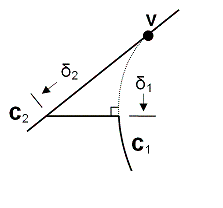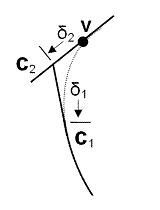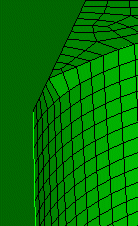Collapse Angle

The collapse command allows the user to collapse small angles using virtual geometry. The command syntax is:

Collapse Angle at Vertex <id range> [angle <degrees>] [Curve <id1> [Arc_length <length>]] [Curve <id2> [Arc_length <length> | Same_size | Perpendicular | Tangent]] [Composite_vertex <angle>] [Preview]

The collapse angle command is used to eliminate small angles at vertices, where curves meet at a tangential point. The command will split each curve at a specified distance (δ1 and δ2 ) as shown in Figure 1, and create two new vertices along those curves. The remaining small angle will be composited into its neighboring surface using virtual geometry. One of two methods may be used for specifying options for the collapse angle command: (1) Simple, and (2) Complete as described below:

(1) Simple

Curves are not specified for this option. Instead, Cubit will automatically identify the smallest angle at the vertex and collapse it using the tangent option described below (see Figure 4). An optional angle option may also be specified that controls the arc length (δ1) along curve C1 where the curve will be split. If not specified a value of 30 degrees will be used. For the simple option of this command, a range of vertices may be used. The complete version of the command requires exactly one vertex and two curves.

(2) Complete

The complete options of the command allow you to specify which curves and where to split each curve. You must input a distance for the first curve ( δ1), but the second location can be determined based on the length and direction of the first curve.Figure 1. Collapse angle syntax

The arclength option will split each curve at a specified distance δ1 and δ2, (See Figure 1) measured from the vertex. You must input at least one arclength for each of the options listed below.

The same_size option will split curve 2 so that the two resulting curves, δ1 and δ2, are the same length as shown in Figure 2.Figure 2. Collapse angle using the same_size option

The perpendicular option will split curve 2 so it is perpendicular to the split location on curve 1, as shown in Figure 3.Figure 3. Collapse angle using the perpendicular option

The tangent option will split curve 2 where a line tangent to curve 1 at the split location intersects curve 2, as shown in Figure 4.Figure 4. Collapse angle using the tangent option

The composite_vertex option automatically composites resulting surfaces if there are only two curves left at the vertex, and the angle is less than a specified tolerance.

The preview option will preview composited surface before applying changes.Figure 5. An example of a meshed surface that is generated after using the collapse angle command.